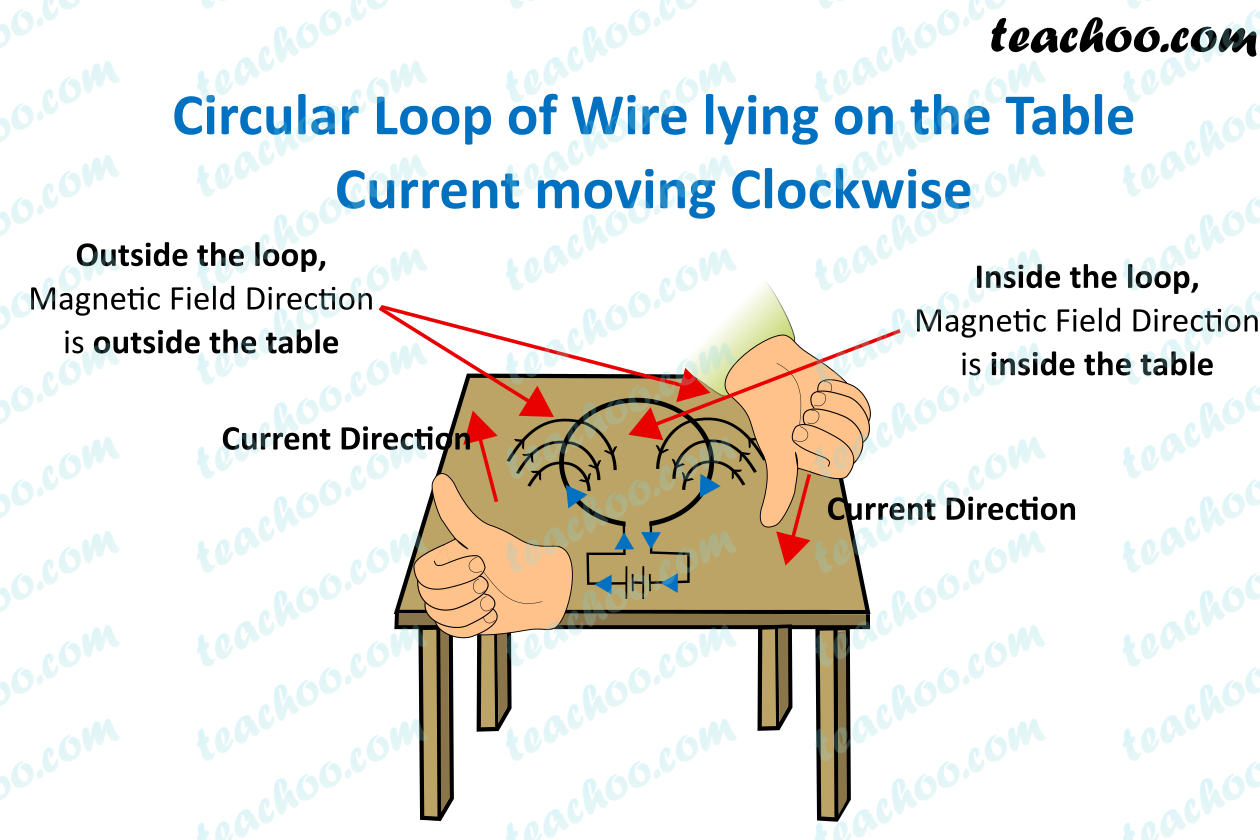## Consider a circular loop of wire lying in the plane of the table. Let the current pass through the loop clockwise. Apply the right-hand rule to find out the direction of the magnetic field inside and outside the loop.We connect the circular wire loop to a battery to ensure the flow of current.

The circular coil is placed in the plane of the table, hence it lies horizontally on the table.

According to the question, the current flows in a clockwise direction.

We can find the direction of magnetic field with the of Maxwell’s right hand thumb rule.

It says that, if we point the thumb of our right hand in the direction of the current

Then the direction in which our fingers curl gives the direction of the current.

If we see the right side of the wire, the current is in downwards direction.

And, If we see the left side of the wire, the current is in upwards direction.

Applying Maxwell’s right hand thumb rule,

We see that inside the loop, the current is into the table

1. Class 10
2. Chapter 13 Class 10 - Magnetic Effects of Electric Current (Term 2)
3. Questions from Inside the chapter

Questions from Inside the chapter

Class 10
Chapter 13 Class 10 - Magnetic Effects of Electric Current (Term 2)